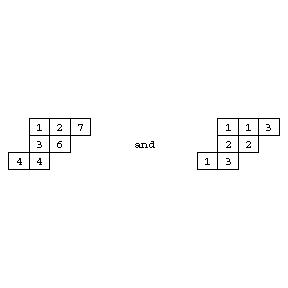Skew Young tableau

Consider two partitions, $\lambda$ of $n+m$ and $\mu$ of $m$. With those partitions one can associate Young diagrams, or Ferrers diagrams (cf. Young diagram), also denoted by $\lambda$ and $\mu$. Suppose that each cell of $\mu$ is also a cell of $\lambda$. The set-difference $\lambda/\mu$ contains exactly $n$ cells. It is called a skew Young diagram, or skew Ferrers diagram.

Such a diagram can be filled with integers from $1$ to $n$ in increasing order in each row and in each column. This is called a standard skew Young tableau. If repetitions are allowed and if the rows are only non-decreasing, the tableau is called semi-standard. These are generalizations of Young tableaux (cf. Young tableau).

For example,Figure: s110150a

are standard, respectively semi-standard, of shape $(4,3,2)/(1,1)$.

It is possible to define the Schur function $S_\lambda(X)$ combinatorially as the generating function of all semi-standard Young tableaux of shape $\lambda$ filled with indices of elements of $X$, as follows.

Let $X=\{x_1,\ldots,x_k\}$ be an alphabet. Let $T$ be a semi-standard Young tableau of shape $\lambda$, containing entries from $1$ to $k$. The product of the indeterminates whose indices appear in $T$ is a monomial. Then $S_\lambda(X)$ is the sum of those monomials over the set of all possible such tableaux $T$.

When replacing Young tableaux by skew Young tableaux of shape $\lambda/\mu$, one obtains the skew Schur function $S_{\lambda/\mu}(X)$. Those functions have many properties in common with ordinary Schur functions. See [a3] for Schur functions.

This connection between combinatorial (Young tableaux) and algebraic (Schur functions) objects is very fruitful, both for combinatorics and for algebra. For applications to $q$-analysis, cf., e.g., [a1] and [a2].